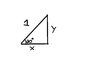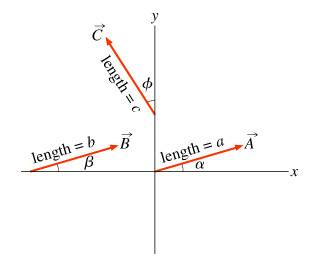# Resolving vector components using trigonometry

NewtonIsaac

## Homework Statement

Find the components of the vector A with length a = 1.00 and angle=20.0° with respect to the x-axis as shown.

Enter the x component followed by the y component, separated by a comma.

none

## The Attempt at a Solution

To find the X-component I did the following:

cos20°=x/1
x=cos20°
x=.408082062

The Y-component was found in a similar fashion:

sin20°=y/1
y=sin20°
y=.912945251

My answer was entered as x,y substituting the relevant values in for x and y. However, the online system I'm using keeps telling me I'm wrong. Can anyone point me in the right direction?

Muphrid
How can the sine of the angle be greater than the cosine? The angle is less than 45 degrees.

NewtonIsaac
I apologize, sometimes I can be mathematically challenged. I don't understand what you are getting at. Here is a picture of my attempt at a solution, in case that helps.

#### Attachments

•problem.jpg
6.8 KB · Views: 722
NewtonIsaac
Here is the actual figure itself.Gold Member
I apologize, sometimes I can be mathematically challenged. I don't understand what you are getting at. Here is a picture of my attempt at a solution, in case that helps.
Your method is correct, it is just that your numbers are wrong. $\cos(45^o) = \sin(45^o)$ and since cos approaches 45 degrees from above and sin from below, you would expect that the cosine of an angle less then 45 degrees be greater than the sine of that angle, as Muphrid stated.

NewtonIsaac
Your method is correct, it is just that your numbers are wrong. $\cos(45^o) = \sin(45^o)$ and since cos approaches 45 degrees from above and sin from below, you would expect that the cosine of an angle less then 45 degrees be greater than the sine of that angle, as Muphrid stated.

Thank you. It's at times like these I feel incredibly stupid. I had my calculator set to radians instead of degrees. Sorry for wasting everyone's time, including my own. Problem solved.

Muphrid
What CAF123 said. It should be apparent just looking at the diagram that it makes no sense for the cosine to be smaller than the sine. The vector is much more in the x-direction than it is in the y-direction.

The reason I point this out is as a general check when doing numerical calculations. It should make you question whether you typed in the numbers to your calculator correctly or if it was in the correct mode. Your angles are in degrees. I suspect you left your calculator in radians.

Edit: good, you figured it out! Leaving the calculator in the wrong angle mode is common, which is why having an intuitive check on what makes sense is really, really useful. That's the only reason I tried to point you in that direction.

NewtonIsaac
What CAF123 said. It should be apparent just looking at the diagram that it makes no sense for the cosine to be smaller than the sine. The vector is much more in the x-direction than it is in the y-direction.

The reason I point this out is as a general check when doing numerical calculations. It should make you question whether you typed in the numbers to your calculator correctly or if it was in the correct mode. Your angles are in degrees. I suspect you left your calculator in radians.

Edit: good, you figured it out! Leaving the calculator in the wrong angle mode is common, which is why having an intuitive check on what makes sense is really, really useful. That's the only reason I tried to point you in that direction.

Yeah, I was actually not aware of how to check that intuitively. More often than not I have found that math does not always come intuitively to me. But now I understand, thank you for your help.